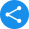# Math

## Learn Basic Maths, Topics and Formulas for Mathematics

Mathematics is that subject which is required at every step of life. A thorough understanding of the concept of basic calculations is required for a student to comprehend his mathematical skills. However, it is seen that children often detest the subject owing to the various formulas, figures and calculations. Studying and teaching mathematics can at times become tiresome but here’s a solution to it. At CoolGyan, mathematics as a subject is made easy to learn and understand. Here, the concepts beginning from the basics to algebra, geometry, trigonometry, arithmetic, calculus, probability and many more are explained with accuracy and in a simple manner to help the students enjoy learning mathematics. Practise sums are also provided to help students learn with clarity and also test their understanding of the concepts.

Mathematics is all about having a strong knowledge of the basics. Hence, topics which give a firm foundation to the knowledge of mathematics of a student are discussed here. These topics include the concepts of Precision, Bodmas Rule, Branches of Mathematics, Cuboid and Cube. Prime Numbers, Divisibility Rules, Set Theory Symbols, Area of Hollow Cylinder, Three Dimensional Shapes, Decimals in Daily Life, Odd Numbers, Algebra Symbols, Geometric Tools, Chance and Probability and many more. Each of these topics is explained and discussed with great precision and elaboration. A proper introduction to every topic is given and all terminologies are well defined. Children learn better with illustrations, and therefore, CoolGyan uses various illustrations and examples to explain the various concepts. At every step appropriate and real-life examples are given thus making it relatable for the student and providing him with a clear picture of whatever he has read or understood so far.

Share this with your friendsShare